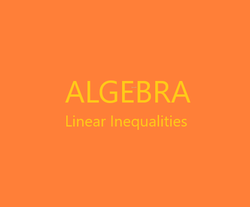# The Retired Professor Simplifies Algebra: Linear Inequalities

## This is the second part of a free resource to help with the basic understqanding of algebra. It can be used by students and those helping students witht the understanding.

The Retired Professor Simplifies is a series of topics designed to be brief presentations that will enable parents to assist in the learning process. The articles can be used directly by the student, or by a parent trying to explain an unfamiliar topic.

This is the first segment of a series that addresses algebra in a simple manner. The first part of the algebra series is linear equations, and it includes absolute value equations and special cases.

The parts of the series will have links to the other parts. Viewing other parts is sometimes necessary, since algebra builds on itself.

### Overcoming Math Anxiety

Before we start it is important to address whether there is a problem with math anxiety.  If there is a belief by the student that it is impossible to learn math, that impediment must be addressed first.  Fortunately, there are some excellent books written on the subject that can really help.

### Linear Inequalities

Linear inequalities are like equations, except that they each use one of the symbols <, >, ≤, or ≥.

They are solved much like equations, with two differences.  The first difference is when an inequality is multiplied or divided on both sides by a negative number, the direction of the inequality changes.

To understand why the above is true take two numbers, say 2 and 4.  Observe, 2 < 4.  Next multiply each side by a negative number, say -3.  Now we get -6 and -12.  Observe, -6 > -12.

The other difference is that while it an equation with the variable solved for makes no difference which side of the equation the variable ends up on.  Observe, x = 3 and 3 = x are equally valid ways of expressing a solution.  In inequalities, the solution set x < 6 is the same as 6 > x.  Notice the direction of the inequality.  Most people prefer the variable on the left, so if you solve with the variable on the right be careful when switching sides.

Now, a few examples.

Solve 5x < 10

Divide both sides by 5.

5x/5 < 10/5

x < 2

Notice any value greater than 2 is a solution, so we refer to the solutions of inequalities as solution sets.

Solve 3(x – 2) < 5x + 8.

Break down the symbols of grouping:  3x + (-6) < 5x + 8

Add 6 and -5x to each side.

3x + (-5x) + (-6) + 6 < 5x + (-5x) + 8 + 6

-2x < 14

Divide both sides by -2.

-2x/(-2) >  14/(-2)

x > -7

Solve for x, 2x + 3 < 2x + 1

2x + (-2x) + 3 < 2x + (-2x) + 1

3 < 1

Notice the variable has disappeared, and what is left is a false statement, so the inequality has no solution.

Solve for x, 2x + 3 > 2x + 1

2x + (-2x) + 3 > 2x + (-2x) + 1

3 > 1

Notice the variable has disappeared, and what is left is a true statement, so the inequality has all real numbers as a solution set.

### Linear Equations

##### Part 1 of the Series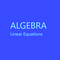Algebra simplified into a brief but thorough free resource can benefit both a parent helping a student and the student. This segment addresses linear equations.

### Absolute Value Inequalities

For linear inequalities we will start with the special cases first, since the special cases give great insight in the process.

First, inequalities of the form │ax + b│ < c where c is 0 or any negative number, or │ax + b│ ≤ d, where d is any negative number and d is any negative number, have no solution.  An absolute value cannot ever be less than 0, so it cannot be less than a negative number.

Next, inequalities of the form │ax + b│ > d, or │ax + b│ ≥ d, where d is any negative number must always be true, so any value of x will solve the inequality.  Hence, the solution set for this case is all real numbers.

The above cases utilize an insight into what an absolute value really is.

Now we consider the more general case.  An absolute value being less than or being less than or equal to a non-negative number.  We get sets of possible solutions, and we must consider only those solutions that make both parts of the set of possible solutions valid.  Hence, we combine the two parts of the solution set with the word “and” which is the same as the intersection of the two sets or answers that we get when solving the inequalities.  Any solution to only one of the two inequalities makes the absolute value inequality less than or at worse equal to a negative value, which is impossible.

As an example, find the solution set for │x + 5│ < 3.

(Many textbooks say to solve x + 5 < 3 and x + 5 > -3.  This does not make clear why the < becomes >.  Our approach makes this more understandable.

Inequality 1 is x + 5 < 3, with solutions x < -2 after subtracting 5 form each side of the inequality.

Inequality 2 is –(x + 5) < 3.  Multiplying each side by -1gives x + 5 > -3.  Notice when we multiplied by a negative number < became >.  So, solving by subtracting 5 from each side of the inequality we get solutions x > -8.

Our solution set is -8 < x < -2.  This is the same as x > -8 “and” x < -2.

Notice x can be negative.  That is fine.

When the absolute value uses the > or ≥ symbol its two possible solution sets is combined using “or” and the solution set is any solution in ether possible solution set.

As another example consider │x - 9│ > 5.  Our two inequalities are –(x – 9) > 5 and x – 9 > 5.

Inequality 1 is x – 9 > 5, which is solved by we add 9 to each side and get x > 14.

Inequality 2 is –(x – 9) > 5.  First, multiply both sides by -1 to produce x – 9 < -5.  Adding 9 to each side we get the solutions x < 4.

The solution set is x < 4 or x > 14.

Note:  The absolute value must be isolated before starting.  Had │x - 9│ - 5 > 0 been the problem │x - 9│ > 5 it would have had to be manipulated into the second form before starting.

If a problem starts with │x - 9│ < -3 simply indicate the problem has no solution.

If a problem starts with │x - 9│ > -3 simply indicate the problem has a solution set of all real numbers.

If a problem starts with │x - 9│ - 8 > -3 add 8 to each side, and solve │x - 9│ > 5 as was done above.  Just seeing the negative number on the right side of the inequality is not enough to make a call of no solution or all real numbers are solutions, the absolute value must first be isolated on one side of the inequality.

### Other Articles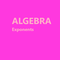This is a free resource on understanding algebra, and presented in a short presentation aimed at making things clear. It may help thoses studying algebra and those trying to help.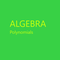This of a free resource where insights into algebra are provided to help both students and those helping students with algebra.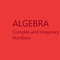This is a free to use algebra resource. It is designed to make it easy to understand complex and imaginary numbers for students and those assisting students with learning.

This article contains links to affiliate programs and Adsense advertising.  These must use cookies to allow for proper crediting, and allow me to earn from qualifying purchases. As an Amazon Associate I earn from qualifying purchases.

Updated: 02/09/2021, blackspanielgallery
1

Only logged-in users are allowed to comment. Login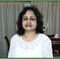WriterArtist on 05/03/2021

Hi blackspanielgallery,
Your way of explaining things are self explanatory. I can see students gaining through your examples. Although I could score good marks in Maths, it was my brother who surpassed everyone.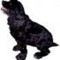blackspanielgallery on 02/09/2021

I hope to go on to calculus, trig, and physics. Since these were ready I started here. I am hoping the Wizzley community accepts the type of article I propose, and other fields will soon be represented. I have single topics, since book length is too long for the platform, and people would need interactive ability to go to topice easily. Here, they can click on the other link, which I have been adding.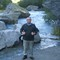frankbeswick on 02/09/2021

There is currently a demand for high school resources, 11-16, but advanced level maths and physics [16-18] will always find readers.blackspanielgallery on 02/08/2021

I have six more that await the intro to be written, a minor thing. They will be coming out soon. And I also plan some physics.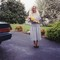DerdriuMarriner on 02/08/2021

blackspanielgallery, Thank you for the practical information and the product line.
It's so enjoyable to go through the non-solution or solution of the equation step-by-step. Perhaps some of the fun results from the former, which I remember as quite delightful in algebra: an inherently unsolvable equation will not be the fault of the solver!
Will you write more on this retired professor simplifies series?

#### You might also like

Learn Algebra and Trigonometry with Rap Videos

Learn the hard to grasp Algebra and Trigonometry basics from inspiring math t...

The Retired Professor Simplifies Algebra: Systems of Equations

Insights into algebra with attention to systems of linear equations is the fo...

Disclosure: This page generates income for authors based on affiliate relationships with our partners, including Amazon, Google and others.